# Optimal Transport vs. Fisher-Rao distance between copulas for clustering multivariate time series

by Gautier MARTI, Hellebore Capital Ltd (joint work with Sébastien ANDLER, research intern at Hellebore Capital)

This article provides the numerical experiments of our IEEE SSP 2016 paper: Optimal Transport vs. Fisher-Rao distance between copulas for clustering multivariate time series. It is also designed to stimulate discussion on this topic at the Geometry in Machine Learning workshop at ICML 2016. The notebook and related code to reproduce experiments is provided for download in a zipped folder at the bottom of this page.

Copulas are distributions which encode the dependence between random variables. Because it is complicated to work with the whole distribution, one usually extracts meaningful numbers from it called correlation / association / dependence coefficients. These numbers only measure particular aspects of the dependence structure: monotone association, tail-dependence, etc. When extracted from copulas that encode a more complex dependence pattern, they can even be misleading. It motivates the need to develop tools to deal with the whole distribution. We may need to:

• compare two dependence structures (distance between two copulas);
• summarize several dependence structures (barycenter of several copulas);
• extract the strength of the association between the variables (projection of the copula onto $[0,1]$).
Basically, we aim at defining a relevant geometry for the space of copulas.

Thus, we focus in this article to determine which distances are relevant between copulas and which are not for these purposes.

• First, we provide an example which highlights an unwanted property shared by a large class of these distances.
• Then, we show how to compute a mean of such distributions according to the Optimal Transport and the Fisher-Rao distances.

# Statistical distances

Statistical distances are distances between probability distributions.

Celebrated ones are the Fisher-Rao geodesic distance and its related $f$-divergences. These distances (e.g. Kullback–Leibler divergence) are often used in signal processing to compare the signal distributions, and, most of the times, improve empirical results.

Another important class of statistical distances is the Optimal Transportation one. It comprises distances that are parameterized by a ground metric, i.e. the metric of the underlying space. The Wasserstein metric is the most known of such distances, but others such as the Sinkhorn distances (entropic regularization of Wasserstein) have came to light recently due to their computational advantage (solved order of magnitudes faster). Unlike the previous divergences, optimal transport distances are well-deﬁned for both discrete (e.g. empirical) and continuous measures.

In the following of this article, we study the behaviour of both distance-classes on the parameter space (with the Gaussian case), and the sample space (with the Student case) of copulas.

#### The Gaussian copula case

When considering Gaussian copulas, we know analytical formulas for the distances. These closed-form expressions are reported in Table 1.

The Gaussian copula is a distribution over the unit cube $[0,1]^d$. It is constructed from a multivariate normal distribution over $\mathbf{R}^d$ by using the probability integral transform. For a given correlation matrix $R \in \mathbf{R}^{d \times d}$, the Gaussian copula with parameter matrix $R$ can be written as $$C_R^{\mathrm{Gauss}}(u_1,\ldots,u_d) = \Phi_R(\Phi^{-1}(u_1),\ldots,\Phi^{-1}(u_d)),$$ where $\Phi^{-1}$ is the inverse cumulative distribution function of a standard normal and $\Phi_R$ is the joint cumulative distribution function of a multivariate normal distribution with mean vector zero and covariance matrix equal to the correlation matrix $R$.

We now illustrate how the distances behave between Gaussian copulas using the following three bivariate Gaussian copulas $C_{R_A}^{\mathrm{Gauss}}, C_{R_B}^{\mathrm{Gauss}}, C_{R_C}^{\mathrm{Gauss}}$, parameterized by $R_A = \begin{pmatrix} 1 & 0.5 \\ 0.5 & 1 \end{pmatrix}, R_B = \begin{pmatrix} 1 & 0.99 \\ 0.99 & 1 \end{pmatrix}, R_C = \begin{pmatrix} 1 & 0.9999 \\ 0.9999 & 1 \end{pmatrix}$ respectively.

In :
from display_utils import *
%matplotlib inline
R_A = [[1,0.5],
[0.5,1]]
R_B = [[1,0.99],
[0.99,1]]
R_C = [[1,0.9999],
[0.9999,1]]
covs = [R_A,R_B,R_C]
display_gaussian_copulas(covs)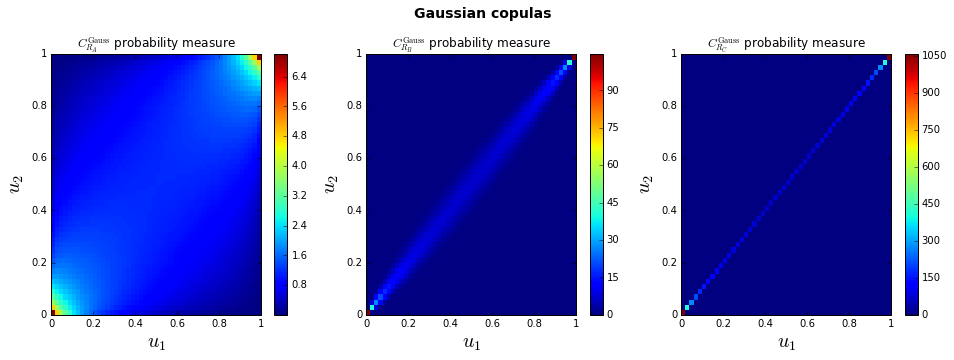We now compute the distances $D(R_A,R_B)$ between $C_{R_A}^{\mathrm{Gauss}}, C_{R_B}^{\mathrm{Gauss}}$ probability measures, and $D(R_B,R_C)$ between $C_{R_B}^{\mathrm{Gauss}}, C_{R_C}^{\mathrm{Gauss}}$ probability measures.

The distance behaviour we expect for clustering the copulas by similar dependence is such that: $$D(R_A,R_B) > D(R_B,R_C).$$

In :
import pandas as pd
from divergences import *
distances = [fisher_rao_GC, KL_GC, Jeffreys_GC, Hellinger_GC, Bhatta_GC, wasserstein_GC]
args = [(0.5,0.99),(0.99,0.9999)]
dist_table = pd.DataFrame([[dist(*arg) for arg in args] for dist in distances],
columns=['$D(R_A,R_B)$','$D(R_B,R_C)$'],
index=['Fisher-Rao','KL$(\Sigma_1\|\Sigma_2)$','Jeffreys','Hellinger','Bhattacharyya','$W_2$'])
dist_table

Out:
$D(R_A,R_B)$ $D(R_B,R_C)$
Fisher-Rao 2.773430 3.256349
KL$(\Sigma_1\|\Sigma_2)$ 22.562208 47.197421
Jeffreys 24.050218 49.005012
Hellinger 0.476025 0.555007
Bhattacharyya 0.646311 0.809696
$W_2$ 0.634939 0.090068Remark. We can observe that unlike Wasserstein $W_2$ distance, Fisher-Rao and related divergences consider that $C_{R_A}^{\mathrm{Gauss}}$ and $C_{R_B}^{\mathrm{Gauss}}$ are nearer than $C_{R_B}^{\mathrm{Gauss}}$ and $C_{R_C}^{\mathrm{Gauss}}$. This may sound surprising since $C_{R_B}^{\mathrm{Gauss}}$ and $C_{R_C}^{\mathrm{Gauss}}$ both describe a strong positive dependence between the two variates whereas $C_{R_A}^{\mathrm{Gauss}}$ describes only a mild positive dependence.

To illustrate how the distances between two bivariate Gaussian copulas vary, we compute the heatmaps of $D\left(\begin{pmatrix} 1 & \rho_1 \\ \rho_1 & 1 \end{pmatrix}, \begin{pmatrix} 1 & \rho_2 \\ \rho_2 & 1 \end{pmatrix} \right)$ for $\rho_1, \rho_2 \in [0,1]$.

In :
display_heatmaps(distances)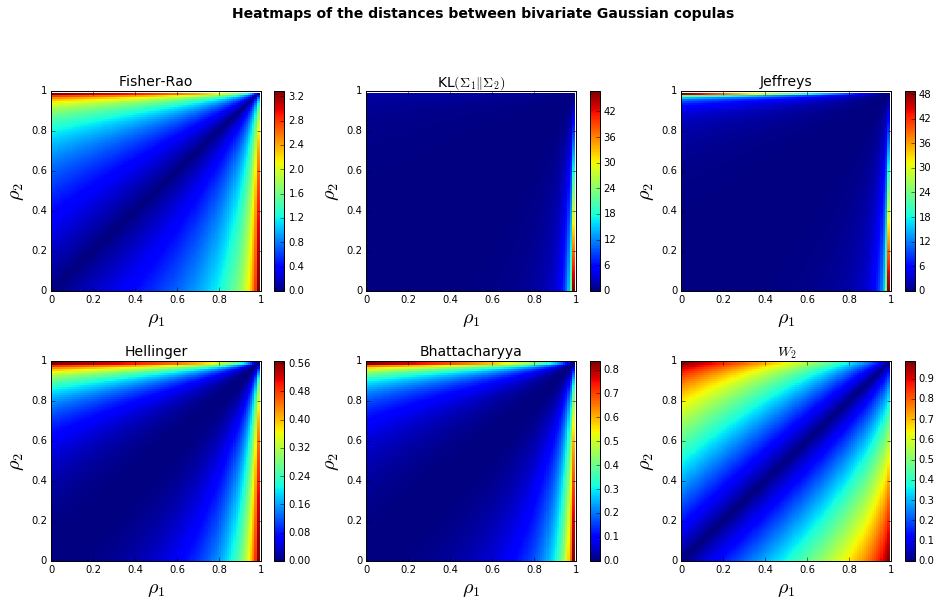Remark. The Fisher-Rao metric curves the space by the 'right amount' to discriminate 'fairly' between the distributions. For example, in the case of correlation, the Cramér-Rao Lower Bound tells us that stronger the correlation, finer the estimate can be: $$\mathrm{Var}(\hat{\rho}) \geq \frac{1}{\mathcal{I}(\rho)} = \frac{\left(1-\rho^2\right)^2}{1+\rho^2},$$ where $\mathcal{I}(\rho)$ is the Fisher Information which is by definition $I(\rho) = -\mathbb{E}\left[\frac{\partial^2}{\partial \rho^2} \log f(X,\rho) \right]$, where $f$ is the bivariate Gaussian density. So, the discriminative power of the Fisher-Rao distance is adjusted to the statistical uncertainty of the correlation estimate.

The related $f$-divergences share partly the same behaviour since they coincide locally with the quadratic form approximations of the Fisher-Rao distance between two close distributions. For example, $KL(\rho\|\rho+d\rho) = \frac{1}{2}\rho I(\rho) \rho$.

Fisher-Rao and its related $f$-divergences are therefore particularly suited to detect precisely and as soon as possible a deviation from a stationary distribution. This property is leveraged, for example, in radar signal processing.

We can notice on the Wasserstein $W_2$ heatmap that its discriminative power is roughly equal for the whole range of values $\rho$. This yields to the clustering behaviour we want for clustering copulas. However, we can also notice that a naive Euclidean $L_2$ distance on the parameter space $\{\rho \in [0,1]\}$ yields to approximately the same behaviour. Why choosing then a complicated optimal transport distance rather than a standard one? We will see in the Barycenter section later on that optimal transport benefits from other attractive properties that $L_2$ does not have.

#### The Student-t copula case

We illustrate the same fact as before, but this time with the Student-t copula, and on the sample space rather than on the parameter space since we do not know closed-form expressions of the distances.

The Student-t copula is a distribution over the unit cube $[0,1]^d$. It is constructed from a multivariate Student's t distribution over $\mathbf{R}^d$ by using the probability integral transform. For a given correlation matrix $R \in \mathbf{R}^{d \times d}$, the Student-t copula with parameter matrix $R$ and degree of freedom $\nu$ can be written as $$C_{R,\nu}^{\mathrm{St-t}}(u_1,\ldots,u_d) = t_{R,\nu}(t_{\nu}^{-1}(u_1),\ldots,t_{\nu}^{-1}(u_d)),$$ where $t_{\nu}^{-1}$ is the inverse cumulative distribution function of a univariate Student's $t$ distribution and $t_{R,\nu}$ is the joint cumulative distribution function of a multivariate Student's $t$ distribution with mean vector zero, degree of freedom $\nu$, and covariance matrix equal to the correlation matrix $R$.

We now illustrate how the distances behave between Student-t copulas using the following three bivariate Student-t copulas $C_{R_A}^{\mathrm{St-t}}, C_{R_B}^{\mathrm{St-t}}, C_{R_C}^{\mathrm{St-t}}$, parameterized as before by $R_A = \begin{pmatrix} 1 & 0.5 \\ 0.5 & 1 \end{pmatrix}, R_B = \begin{pmatrix} 1 & 0.99 \\ 0.99 & 1 \end{pmatrix}, R_C = \begin{pmatrix} 1 & 0.9999 \\ 0.9999 & 1 \end{pmatrix}$ respectively.

In the case of Student-t copulas, we do not know analytical expressions for the distances in the parameter space, so we will illustrate their behaviour on the sample space. Below the expression of the distances:

• $f$-divergences:
• Kullback-Leibler divergence: $KL(p\|q) = \sum_i p(i) \log \frac{p(i)}{q(i)}$
• Jeffreys divergence: $J(p,q) = KL(p\|q) + KL(q\|p)$
• Hellinger distance: $H(p,q) = \frac{1}{\sqrt{2}} \sqrt{\sum_i \left( \sqrt{p(i)} - \sqrt{q(i)} \right)^2}$
• Bhattacharyya distance: $B(p,q) = - \log \sum_i \sqrt{p(i)q(i)}$
• optimal transportation distances:
• Earth mover's distance: $\mathrm{EMD}(p,q) = \min_{P \in U(p,q)} \langle P, M \rangle$, $U$ the transporation polytope, $M$ the ground metric
• Sinkhorn distance: $S(p,q) = \min_{P \in U_{\alpha}(p,q)} \langle P, M \rangle$, $U_{\alpha}$ a Kullback-Leibler ball of radius $\alpha$ inside $U$

We do not compute EMD in the following due to its prohibitive computational cost, but it is implemented in divergences.py as emdist if needed.

In :
distances = [KL, Jeffreys, Hellinger, Bhatta, sinkhorn]
dofs = [3,150]
display_student_copulas(covs,dofs,distances)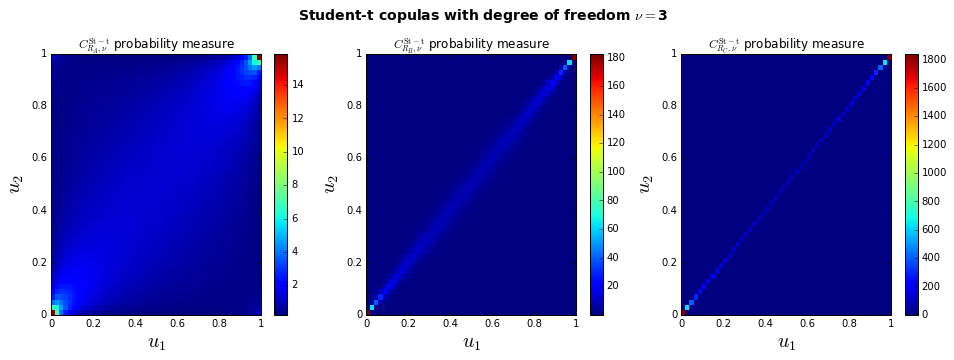$D(R_A,R_B)$ $D(R_B,R_C)$
KL 2.832211 3.622727
Jeffreys 4.205717 4.514926
Hellinger 0.626341 0.577275
Bhattacharyya 0.498079 0.405335
Sinkhorn 0.048834 0.030344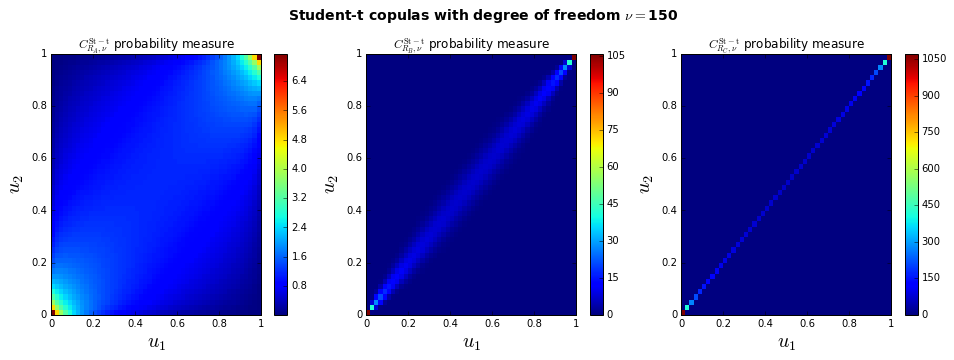$D(R_A,R_B)$ $D(R_B,R_C)$
KL 16.045267 inf
Jeffreys 17.557622 NaN
Hellinger 0.690854 0.664818
Bhattacharyya 0.648708 0.583366
Sinkhorn 0.042363 0.027648

Remark. We notice the same unwanted behaviour for the $f$-divergences on the sample space. Besides, KL and Jeffreys suffer from numerical precision issues (very small density values are put to 0). If we work with empirical distributions (a set of discrete measures built from observation), one has to carefully preprocess the measures with a kernel density estimator before computing the divergences in order to avoir this numerical issue. So much troubles that are easily avoided by using an optimal transport distance, which in addition behaves well.

# Barycenters

Question. What is the mean correlation?

For computing a mean correlation from a set of $N$ copulas, should we:

• Extract $N$ correlation coefficients from the $N$ copulas, and then compute a mean of the $N$ correlation coefficients?
• or
• Compute the mean of the $N$ copulas, and then eventually extract a correlation coefficient from the mean copula?

Given several copulas, we may want to summarize these distributions, i.e. computing a 'mean' copula.

There exist several types of means which usually depend on the distance chosen. The Fréchet mean is a generalization of centroids to metric spaces.

Fréchet Mean. Let $x_1,\ldots,x_N$ be $N$ points in a metric space $(M,d)$. The Fréchet mean is the point $m$ of $M$ such that $$m = \mathrm{argmin}_{p \in M} \sum_{i=1}^N d^2(p,x_i).$$

First, we illustrate the difference between the Fréchet mean for Fisher-Rao geodesic distance and the Fréchet mean for Wasserstein $W_2$ on Gaussians. Then, we will compute the Fréchet means for arbitrary and empirical distributions using the (optimal transport) Sinkhorn distances.

### The Gaussian case

Example with Gaussian copulas. Let's consider 10 bivariate Gaussian copulas with correlations $0.2,0.2,0.25,0.3,0.3,0.3,0.5,0.7,0.7,0.9$ respectively. In the following, we will compute the Fisher-Rao Fréchet mean and Wasserstein $W_2$ Fréchet mean of these $10$ correlation matrices which live in the correlation matrices submanifold of the covariance matrices manifold. We deduce from the mean correlation matrix the associated Gaussian mean copula parameterized by this mean correlation matrix.

Let $\{C^{(k)}\}_k$ be Gaussian copulas parameterized with correlation matrices $\{R^{(k)}\}$. The Fréchet Mean of $\{R^{(k)}\}_k$ is $R^\star$ so that $$R^\star = \mathrm{argmin}_R \sum_{k=1}^N d^2(R,R^{(k)}).$$

In :
corrs = [0.2,0.2,0.25,0.3,0.3,0.3,0.5,0.7,0.7,0.9]
covs = [[[1,corr],[corr,1]] for corr in corrs]

print("For input correlations:\n\nArithmetic mean: "+str(round(np.mean(corrs),2))\
+"\nGeometric mean: "+str(round(scipy.stats.mstats.gmean(corrs),2))\
+"\nHarmonic mean: "+str(round(scipy.stats.mstats.hmean(corrs),2)))

For input correlations:
Arithmetic mean: 0.44
Geometric mean: 0.38
Harmonic mean: 0.33


Fréchet Mean of Covariances for Fisher-Rao. Let's take $d =$ Fisher-Rao. We can express it as $$d^2(R,R^{(k)}) = \left\langle \log\left(R^{-1/2} R^{(k)} R^{-1/2}\right), \log\left(R^{-1/2} R^{(k)} R^{-1/2}\right) \right\rangle.$$

We can find the minimizer of this convex optimization problem thanks to a steepest gradient descent with step size $\alpha \in ]0,1[$: $$R_{n+1} = R_n^{1/2} \exp\left( \alpha \sum_{k=1}^N \log\left(R_n^{-1/2} R^{(k)} R_n^{-1/2}\right) \right) R_n^{1/2}.$$

In :
from barycenters import *
mean, proj_mean = compute_fisher_rao_gaussian_barycenter(covs)
print("mean:\n",mean,"\n\n","projected mean:\n",proj_mean)

mean:
[[ 0.9515503   0.46517133]
[ 0.46517133  0.9515503 ]]
projected mean:
[[ 1.          0.48885627]
[ 0.48885627  1.        ]]

In :
display_gaussian_copula(proj_mean,'Mean Gaussian Copula for Fisher-Rao')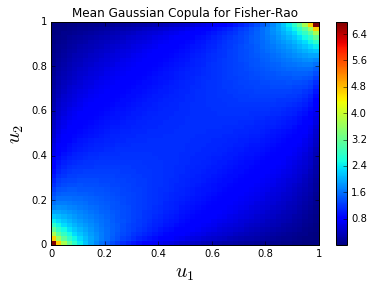Remark. Notice that the mean of correlation matrices is a covariance matrix. It means that the correlation matrices submanifold is not totally geodesic in the covariance matrices manifold. We recover a correlation matrix by rescaling the variances, i.e. $\mathrm{diag}(R^\star)^{-1/2} R^\star \mathrm{diag}(R^\star)^{-1/2}$.

Question: How can we stay in the submanifold during the optimization? That is searching the solution $R^\star$ in the elliptope $E = \{R \in \mathbb{R}^{n \times n}~|~ R = R^\top; R_{ii} = 1, i=1,\ldots,n; R \succeq 0 \}$ endowed with the Fisher-Rao Riemannian metric: $$R^\star = \mathrm{argmin}_{R \in E} \sum_{k=1}^N d^2(R,R^{(k)}).$$

Fréchet Mean of Covariances for Wasserstein $W_2$. Let's take $d =$ Wasserstein $W_2$. We can express it as $$d^2(R,R^{(k)}) = tr\left(R + R^{(k)} -2\sqrt{R^{1/2} R^{(k)} R^{1/2}} \right).$$

We can find the minimizer thanks to Theorem 4.2 eq. (19) from A fixed-point approach to barycenters in Wasserstein space: $$R_{n+1} = R_{n}^{-1/2} \left( \sum_{k=1}^{N} \left( R_{n}^{1/2} R^{(k)} R_{n}^{1/2} \right)^{1/2} \right)^2 R_{n}^{-1/2}.$$

In :
mean, proj_mean = compute_wasserstein_gaussian_barycenter(covs)
print("mean:\n",mean,"\n\n","projected mean:\n",proj_mean)

mean:
[[ 97.81085998  44.75945522]
[ 44.75945522  97.81085998]]
projected mean:
[[ 1.          0.45761233]
[ 0.45761233  1.        ]]

In :
display_gaussian_copula(proj_mean,'Mean Gaussian Copula for Wasserstein $W_2$')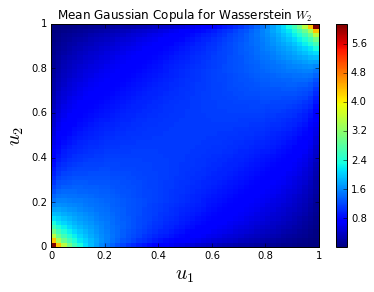Remark. For the same reason, same remark as before: The correlation matrices submanifold is not totally geodesic.

We can also notice that in both cases, the correlation of the mean copula is higher than the mean of correlation values. This point needs to be further investigated: It could mean that describing the average pairwise correlation of a portfolio with a naive mean of the correlation coefficients could underestimate the 'true' average pairwise correlation.

Another interesting question that is raised here: Can we compute or approximate the correlation of the mean copula with some generalized mean computed on the set of correlation coefficients?

### The empirical case

Fréchet Mean of empirical copulas. When working with empirical measures, computing these centers becomes more involved: Divergences are usually ill-defined; Exact OT scales in $O(\#diracs^3)$ for a single distance computation. To be able to compute barycenters effectively, one has to choose either a naive distance such as $L_2$ (at one's own risk), or performing kernel density estimators for smoothing the empirical measures before using divergences (yet the barycenter may be skewed toward strong correlation as shown in the first part of this article), or efficient computational scheme for approximating the optimal transport in a reasonable amount of time.

We show below (with a specific dependence pattern) the benefit of using an optimal transportation barycenter rather than naive or divergence ones which are not 'aware' of the support geometry. Taking into account the support geometry is particularly important when working on real data where dependence pattern can be misaligned due to some noise.

Below we generate 5 copulas describing the dependence between $X \sim \mathcal{U}([0,1])$ and $Y \sim (X \pm \epsilon_i)^2$, where $\epsilon_i$ is a constant noise specific for each distribution. $X$ and $Y$ are counter-monotonic (more or less) half of the time, and co-monotonic (more or less) half of the time.

In :
copulas, nbBins = display_dependence_patterns()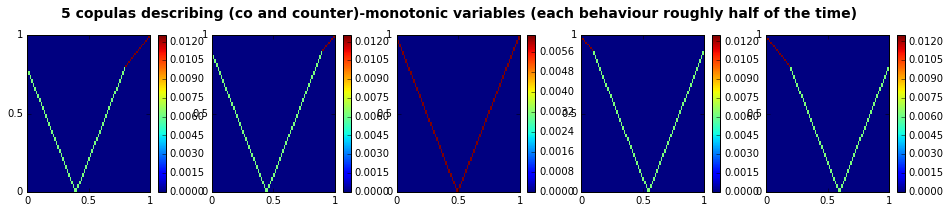We compute and display below the barycenter of the 5 copulas for the $L_2$ geometry and the Wasserstein geometry (using Cuturi's Sinkhorn distance).

In :
lmda = 2650
gamma = 1/lmda
dists = getEuclideanCostFunction(copulas,copulas)
K = np.exp(-dists/gamma)
C = np.zeros((nbBins*nbBins,len(copulas)))
for i in range(len(copulas)):
C[:,i] = copulas[i].reshape(nbBins*nbBins)

centers = [L2_barycenter(C),wasserstein_barycenter(C,K)]

In :
display_barycenters(centers)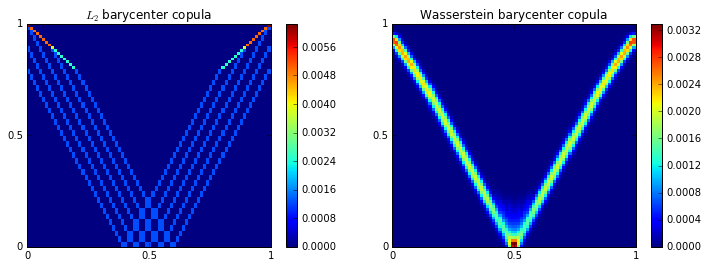Notice that the Wasserstein barycenter better describes the underlying dependence between $X$ and $Y$: the copula encodes a functional association. This is not the case for the Euclidean barycenter.

Discussion

For the task of clustering similar dependence patterns, we may prefer OT geometry, especially when working with (noisy) empirical distributions or with variables which are strongly dependent. Fisher-Rao and the $f$-divergences are better suited when there is a need to incorporate statistical uncertainty of the estimates to calibrate how much two distributions should diverge. This is why they have been so successful in radar signal processing where one aims at detecting as precisely as possible when a real target enters in the radar scope: the distribution changes, but does it change (or diverge) enough with respect to the statistical uncertainty mixed with the ambient noise? Fisher-Rao is a relevant tool to answer this question. However, for the task of clustering similar dependence patterns, Fisher-Rao and the $f$-divergences exhibit:

• an unwanted behaviour,
• numerical instability,
• blindness to the support geometry.